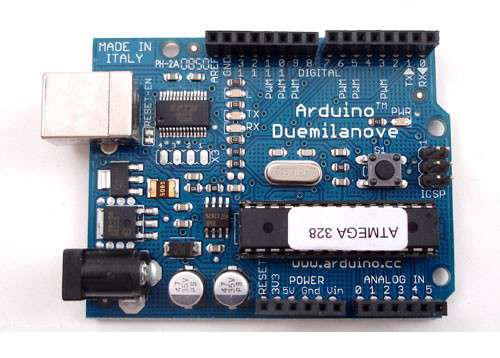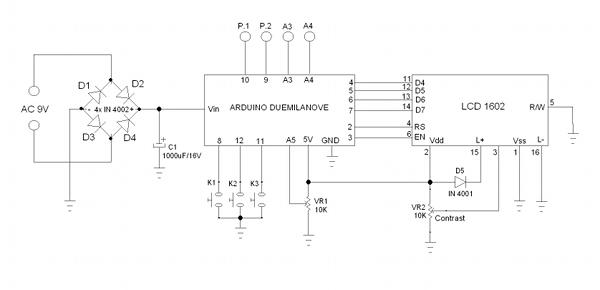# Digital Control for Laboratory Power Supply Using ArduinoFigure 1. Arduino Duemilanove Board for Power Supply’s Digital Control

Digital System for Display and Control

As the second part of the first article Adjustable 1-30V Laboratory Power Supply, the digital control and display for our power supply will be presented here. The microcontroller used in the circuit is ATMEGA 328, a member of Atmel’s AVR microcontroller family. We use Arduino Duemilanove board to ease the development, and you can see the board  in the Figure 1. If you don’t want to use Arduino board then you can make your own microcontroller board based on Arduino system configuration at arduino-duemilanove-schematic.pdf (you can omit many parts which is not needed for this design). Here is the circuit’s schematic diagram employing the Arduino board:Figure 2. Shcematic Diagram of The Digital Control Circuit

The Control and Display Circuit Design

The Program Source Code (The Firmware)

The source code for the arduino system is shown below, how the code works and how to tweak this firmware will be presented in the next update, coming soon!

``` #include <LiquidCrystal.h> #include <stdlib.h>```

LiquidCrystal lcd(2,3,4,5,6,7);
int voltagePin = A3;
int currentPin = A4;
int pwmCurrentPin = 10;
int pwmVoltagePin = 9;
int potPin = A5;
int key1Pin = 8;
int key2Pin = 12;
int key3Pin = 11;
int prevk1=1;
int prevk2=1;
int prevk3=1;
int currk1=1;
int currk2=1;
int currk3=1;
float voltageRef = 0;
float currentRef = 0;

{
float total=0;
for(int n=0;n<100;n++)
{
temp = 1.1 * temp/1024 * 30;
total = total + temp;
delay(1);
}
}

{
float total=0;
for(int n=0;n<100;n++)
{
temp = temp/1024 * 5;
temp = (temp-2.51)*25*0.185;
total = total + temp;
delay(1);
}
}

{
temp = temp/1024;
return temp;
}

void showCurrentSet(float value)
{
char txt;
dtostrf(value,4,1,txt);
lcd.setCursor(11,0);
lcd.print(txt);
}

void showVoltageSet(float value)
{
char txt;
dtostrf(value,5,1,txt);
lcd.setCursor(4,0);
lcd.print(txt);
}

void showVoltageAct(float value)
{
char txt;
dtostrf(value,5,1,txt);
lcd.setCursor(4,1);
lcd.print(txt);
}

void showCurrentAct(float value)
{
char txt;
dtostrf(value,4,1,txt);
lcd.setCursor(11,1);
lcd.print(txt);
}

void setup()
{
lcd.begin(16, 2);
lcd.setCursor(0,0);
lcd.print(“SET:00.00V 0.00A”);
lcd.setCursor(0,1);
lcd.print(“ACT:00.00V 0.00A”);
pinMode(key1Pin, INPUT_PULLUP);
pinMode(key2Pin, INPUT_PULLUP);
pinMode(key3Pin, INPUT_PULLUP);
pinMode(pwmVoltagePin, OUTPUT);
analogWrite(pwmVoltagePin, 0);
pinMode(pwmCurrentPin, OUTPUT);
currentRef = 0.5;
analogWrite(pwmCurrentPin,128 + (currentRef*12.7));
}

void loop()
{
int count = 0;
while (count < 10)
{
if(tempk1 != currk1)
{
tempk1 = currk1;
count = 0;
}
if(tempk2 != currk2)
{
tempk2 = currk2;
count = 0;
}
if(tempk3 != currk3)
{
tempk3 = currk3;
count = 0;
}
count++;
}

if((currk1==0)&&(prevk1==1))
{
currentRef = currentRef + 0.5;
if(currentRef>3) currentRef=3;
showCurrentSet(currentRef);
analogWrite(pwmCurrentPin,128 + (currentRef*11));
}

if((currk2==0)&&(prevk2==1))
{
currentRef = currentRef – 0.5;
if(currentRef<0.5) currentRef=0.5;
showCurrentSet(currentRef);
analogWrite(pwmCurrentPin,128 + (currentRef*12.7));
}

if((currk3==0)&&(prevk3==1))
{
}

prevk1=currk1;
prevk2=currk2;
prevk3=currk3;

showCurrentSet(currentRef);
}

See the software in action, in our Youtube channel below:

•Lanre Johnson

I must share it. It is working, you shorted p&minus output, that means it has some protection.

Show the full schematic or of the PSU sir.

Good and great work. Well done.

•Lanre Johnson

Please, I like to use Atmega 328 nano, with oled 0.96 4pin,128by64 chip: 1306

OR

TZT TFT Display 0.96 / 1.3 inch IPS 7P SPI HD 65K Full Color LCD Module ST7735 Drive IC 80*160 (Not OLED) For Arduino

QUESTION: HOW DO I MODIFY THE CIRCUIT AND .INOSFTWARE FILE HERE? HELP!!
#include
#include

LiquidCrystal lcd(2,3,4,5,6,7);
int voltagePin = A3;
int currentPin = A4;
int pwmCurrentPin = 10;
int pwmVoltagePin = 9;
int potPin = A5;
int key1Pin = 8;
int key2Pin = 12;
int key3Pin = 11;
int prevk1=1;
int prevk2=1;
int prevk3=1;
int currk1=1;
int currk2=1;
int currk3=1;
float voltageRef = 0;
float currentRef = 0;

{
float total=0;
for(int n=0;n<100;n++)
{
temp = 1.1 * temp/1024 * 30;
total = total + temp;
delay(1);
}
}

{
float total=0;
for(int n=0;n<100;n++)
{
temp = temp/1024 * 5;
temp = (temp-2.51)*25*0.185;
total = total + temp;
delay(1);
}
}

{
temp = temp/1024;
return temp;
}

void showCurrentSet(float value)
{
char txt;
dtostrf(value,4,1,txt);
lcd.setCursor(11,0);
lcd.print(txt);
}

void showVoltageSet(float value)
{
char txt;
dtostrf(value,5,1,txt);
lcd.setCursor(4,0);
lcd.print(txt);
}

void showVoltageAct(float value)
{
char txt;
dtostrf(value,5,1,txt);
lcd.setCursor(4,1);
lcd.print(txt);
}

void showCurrentAct(float value)
{
char txt;
dtostrf(value,4,1,txt);
lcd.setCursor(11,1);
lcd.print(txt);
}

void setup()
{
lcd.begin(16, 2);
lcd.setCursor(0,0);
lcd.print(“SET:00.00V 0.00A”);
lcd.setCursor(0,1);
lcd.print(“ACT:00.00V 0.00A”);
pinMode(key1Pin, INPUT_PULLUP);
pinMode(key2Pin, INPUT_PULLUP);
pinMode(key3Pin, INPUT_PULLUP);
pinMode(pwmVoltagePin, OUTPUT);
analogWrite(pwmVoltagePin, 0);
pinMode(pwmCurrentPin, OUTPUT);
currentRef = 0.5;
analogWrite(pwmCurrentPin,128 + (currentRef*12.7));
}

void loop()
{
int count = 0;
while (count 3) currentRef=3;
showCurrentSet(currentRef);
analogWrite(pwmCurrentPin,128 + (currentRef*11));
}

if((currk2==0)&&(prevk2==1))
{
currentRef = currentRef – 0.5;
if(currentRef<0.5) currentRef=0.5;
showCurrentSet(currentRef);
analogWrite(pwmCurrentPin,128 + (currentRef*12.7));
}

if((currk3==0)&&(prevk3==1))
{
}

prevk1=currk1;
prevk2=currk2;
prevk3=currk3;